# Selina Solutions Concise Maths Class 7 Chapter 8: Percent and Percentage Exercise 8C

Selina Solutions Concise Maths Class 7 Chapter 8 Percent and Percentage Exercise 8C explains the concept of percentage change. The solved examples before the exercise problems help students understand the method of solving problems, with ease. The solutions PDF has answers in a stepwise manner based on the latest syllabus of ICSE board. It provides a chance to students to analyse their weaknesses and work on them for a better academic performance. Students can clarify their doubts using Selina Solutions Concise Maths Class 7 Chapter 8 Percent and Percentage Exercise 8C PDF, from the links which are provided below for free download.

## Selina Solutions Concise Maths Class 7 Chapter 8: Percent and Percentage Exercise 8C Download PDF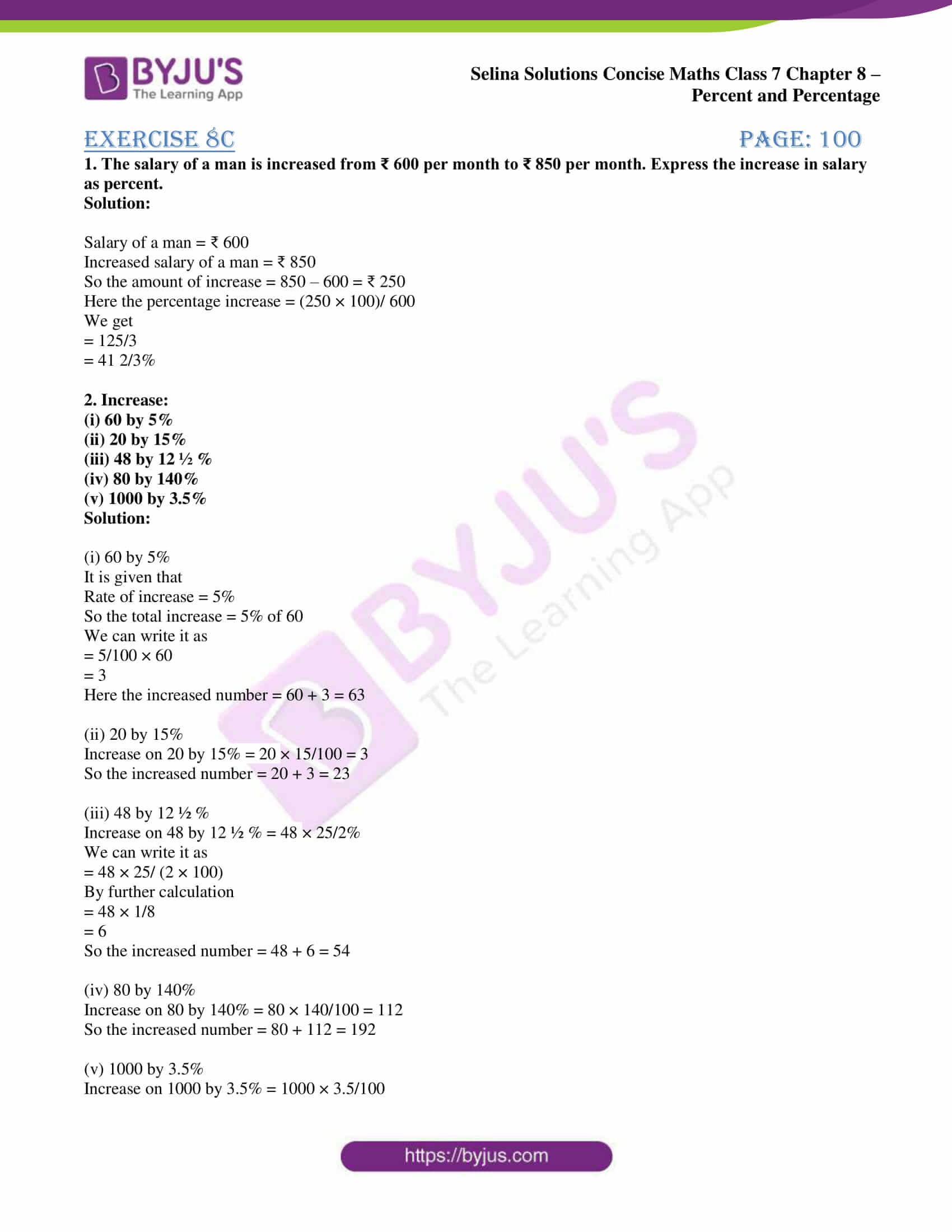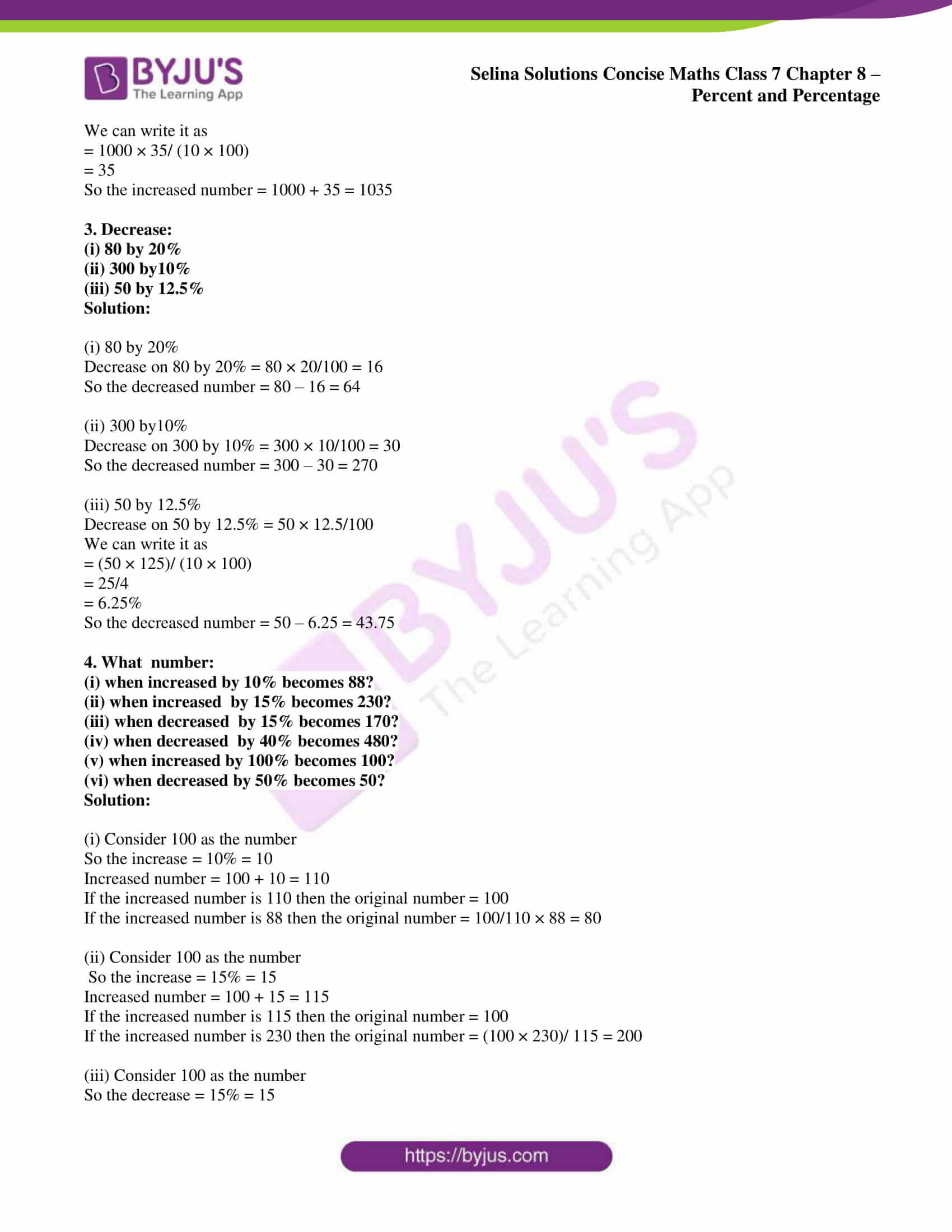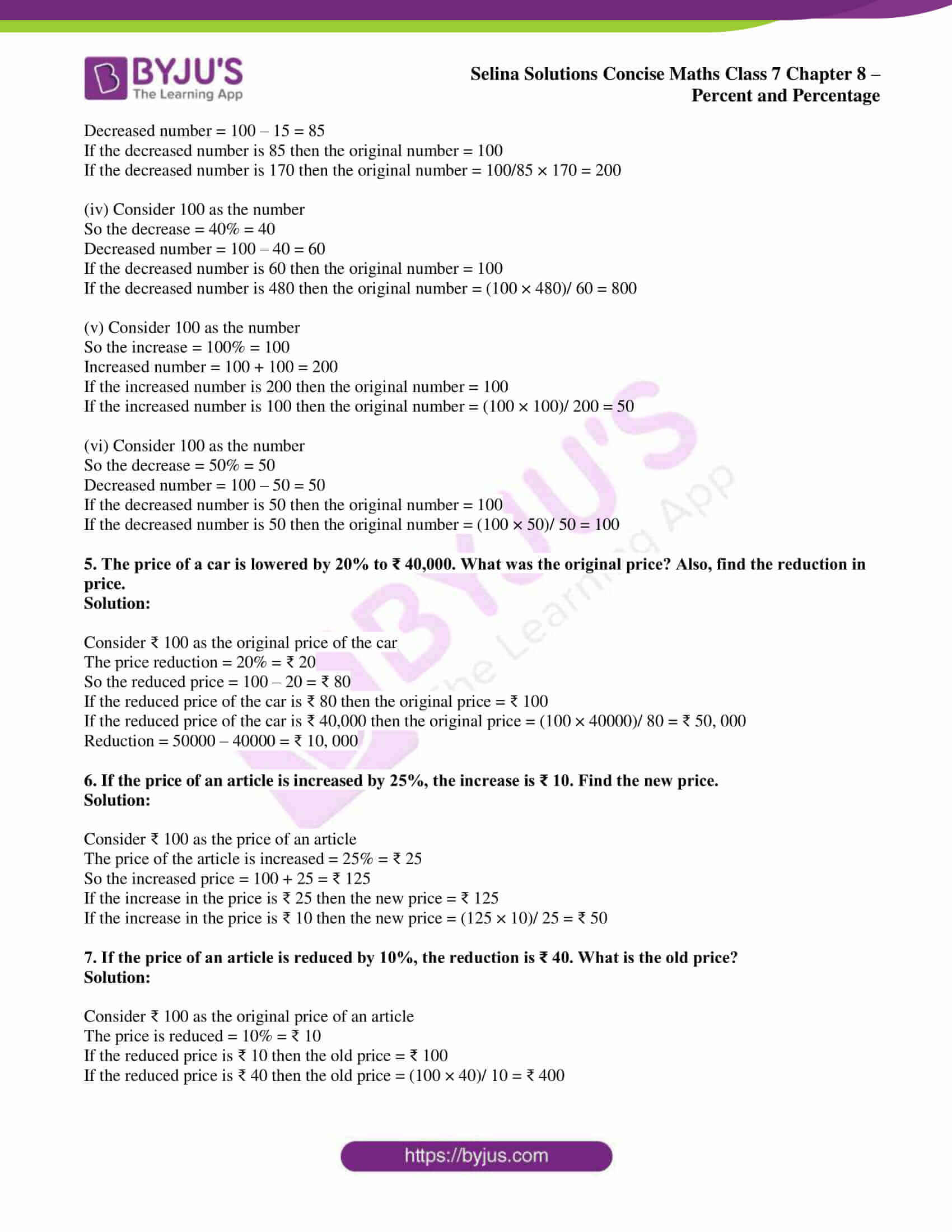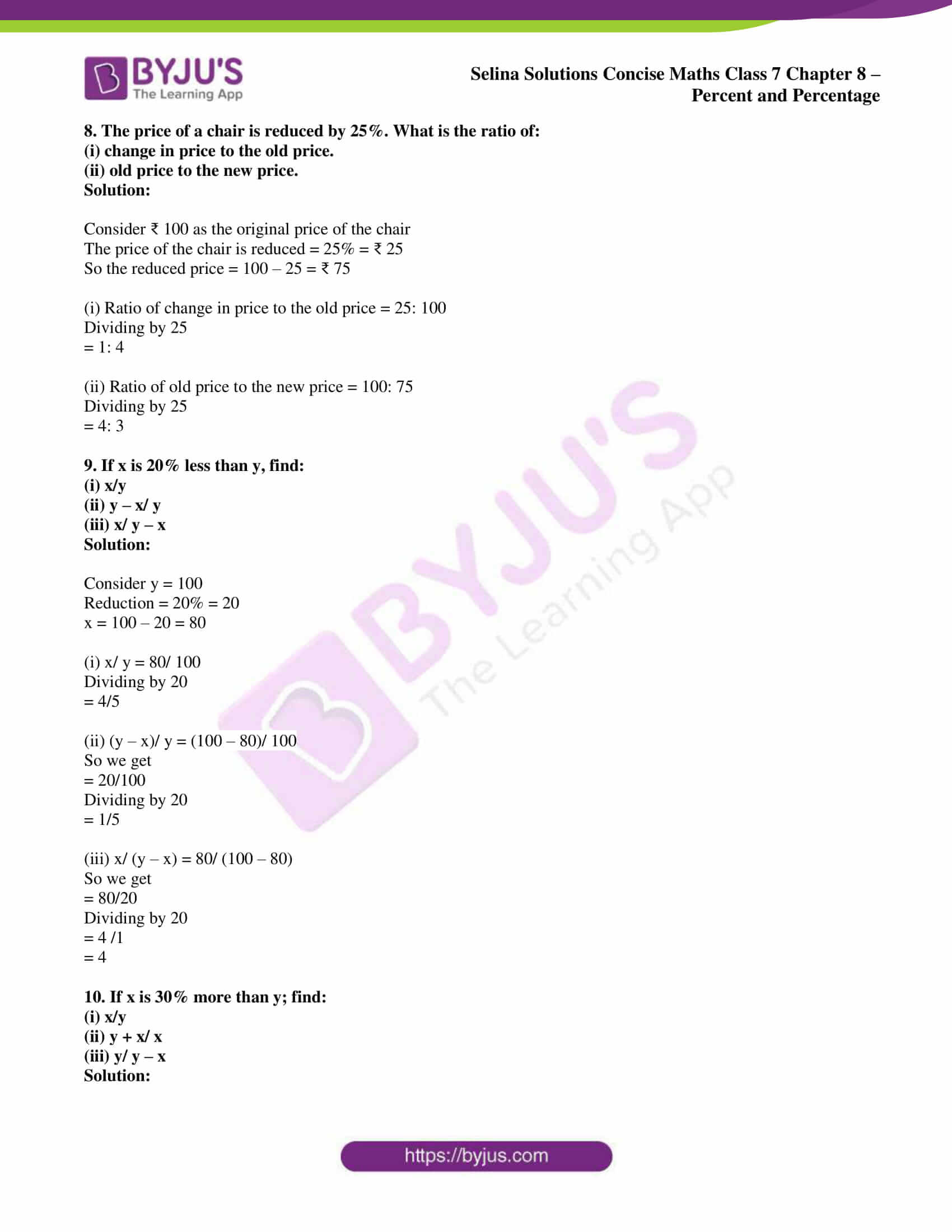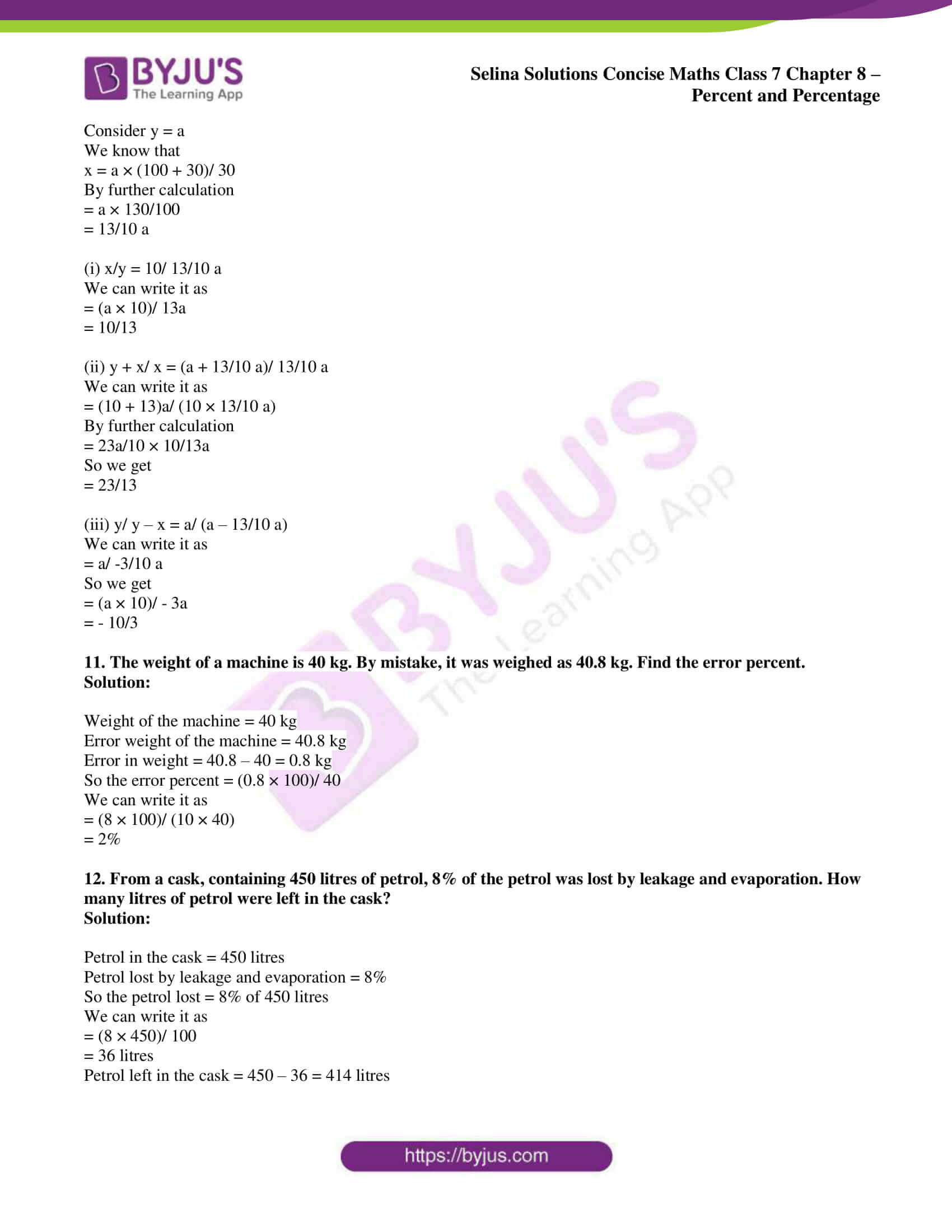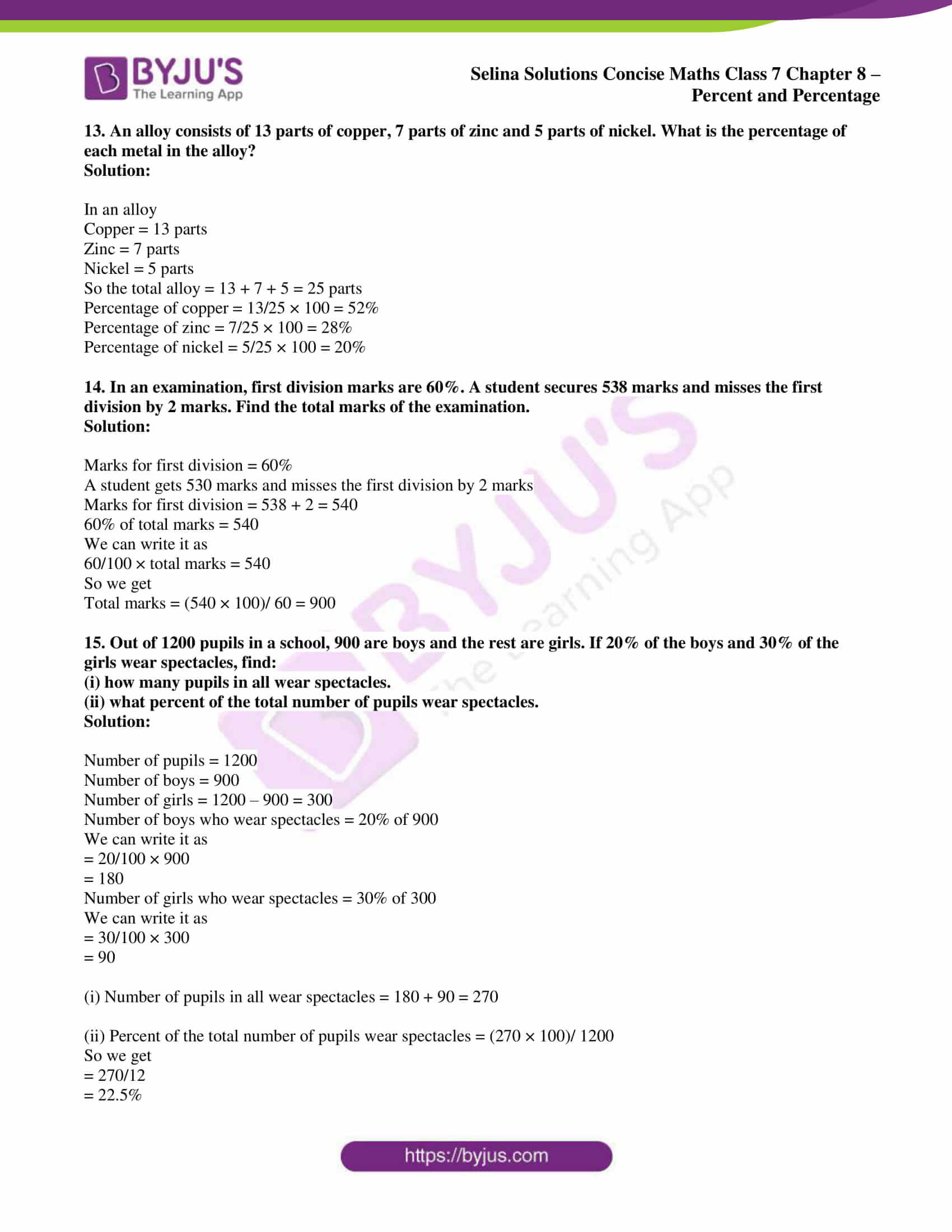### Access Selina Solutions Concise Maths Class 7 Chapter 8: Percent and Percentage Exercise 8C

#### Exercise 8C page: 100

1. The salary of a man is increased from ₹ 600 per month to ₹ 850 per month. Express the increase in salary as percent.

Solution:

Salary of a man = ₹ 600

Increased salary of a man = ₹ 850

So the amount of increase = 850 – 600 = ₹ 250

Here the percentage increase = (250 × 100)/ 600

We get

= 125/3

= 41 2/3%

2. Increase:

(i) 60 by 5%

(ii) 20 by 15%

(iii) 48 by 12 ½ %

(iv) 80 by 140%

(v) 1000 by 3.5%

Solution:

(i) 60 by 5%

It is given that

Rate of increase = 5%

So the total increase = 5% of 60

We can write it as

= 5/100 × 60

= 3

Here the increased number = 60 + 3 = 63

(ii) 20 by 15%

Increase on 20 by 15% = 20 × 15/100 = 3

So the increased number = 20 + 3 = 23

(iii) 48 by 12 ½ %

Increase on 48 by 12 ½ % = 48 × 25/2%

We can write it as

= 48 × 25/ (2 × 100)

By further calculation

= 48 × 1/8

= 6

So the increased number = 48 + 6 = 54

(iv) 80 by 140%

Increase on 80 by 140% = 80 × 140/100 = 112

So the increased number = 80 + 112 = 192

(v) 1000 by 3.5%

Increase on 1000 by 3.5% = 1000 × 3.5/100

We can write it as

= 1000 × 35/ (10 × 100)

= 35

So the increased number = 1000 + 35 = 1035

3. Decrease:

(i) 80 by 20%

(ii) 300 by10%

(iii) 50 by 12.5%

Solution:

(i) 80 by 20%

Decrease on 80 by 20% = 80 × 20/100 = 16

So the decreased number = 80 – 16 = 64

(ii) 300 by10%

Decrease on 300 by 10% = 300 × 10/100 = 30

So the decreased number = 300 – 30 = 270

(iii) 50 by 12.5%

Decrease on 50 by 12.5% = 50 × 12.5/100

We can write it as

= (50 × 125)/ (10 × 100)

= 25/4

= 6.25%

So the decreased number = 50 – 6.25 = 43.75

4. What number:

(i) when increased by 10% becomes 88?

(ii) when increased by 15% becomes 230?

(iii) when decreased by 15% becomes 170?

(iv) when decreased by 40% becomes 480?

(v) when increased by 100% becomes 100?

(vi) when decreased by 50% becomes 50?

Solution:

(i) Consider 100 as the number

So the increase = 10% = 10

Increased number = 100 + 10 = 110

If the increased number is 110 then the original number = 100

If the increased number is 88 then the original number = 100/110 × 88 = 80

(ii) Consider 100 as the number

So the increase = 15% = 15

Increased number = 100 + 15 = 115

If the increased number is 115 then the original number = 100

If the increased number is 230 then the original number = (100 × 230)/ 115 = 200

(iii) Consider 100 as the number

So the decrease = 15% = 15

Decreased number = 100 – 15 = 85

If the decreased number is 85 then the original number = 100

If the decreased number is 170 then the original number = 100/85 × 170 = 200

(iv) Consider 100 as the number

So the decrease = 40% = 40

Decreased number = 100 – 40 = 60

If the decreased number is 60 then the original number = 100

If the decreased number is 480 then the original number = (100 × 480)/ 60 = 800

(v) Consider 100 as the number

So the increase = 100% = 100

Increased number = 100 + 100 = 200

If the increased number is 200 then the original number = 100

If the increased number is 100 then the original number = (100 × 100)/ 200 = 50

(vi) Consider 100 as the number

So the decrease = 50% = 50

Decreased number = 100 – 50 = 50

If the decreased number is 50 then the original number = 100

If the decreased number is 50 then the original number = (100 × 50)/ 50 = 100

5. The price of a car is lowered by 20% to ₹ 40,000. What was the original price? Also, find the reduction in price.

Solution:

Consider ₹ 100 as the original price of the car

The price reduction = 20% = ₹ 20

So the reduced price = 100 – 20 = ₹ 80

If the reduced price of the car is ₹ 80 then the original price = ₹ 100

If the reduced price of the car is ₹ 40,000 then the original price = (100 × 40000)/ 80 = ₹ 50, 000

Reduction = 50000 – 40000 = ₹ 10, 000

6. If the price of an article is increased by 25%, the increase is ₹ 10. Find the new price.

Solution:

Consider ₹ 100 as the price of an article

The price of the article is increased = 25% = ₹ 25

So the increased price = 100 + 25 = ₹ 125

If the increase in the price is ₹ 25 then the new price = ₹ 125

If the increase in the price is ₹ 10 then the new price = (125 × 10)/ 25 = ₹ 50

7. If the price of an article is reduced by 10%, the reduction is ₹ 40. What is the old price?

Solution:

Consider ₹ 100 as the original price of an article

The price is reduced = 10% = ₹ 10

If the reduced price is ₹ 10 then the old price = ₹ 100

If the reduced price is ₹ 40 then the old price = (100 × 40)/ 10 = ₹ 400

8. The price of a chair is reduced by 25%. What is the ratio of:

(i) change in price to the old price.

(ii) old price to the new price.

Solution:

Consider ₹ 100 as the original price of the chair

The price of the chair is reduced = 25% = ₹ 25

So the reduced price = 100 – 25 = ₹ 75

(i) Ratio of change in price to the old price = 25: 100

Dividing by 25

= 1: 4

(ii) Ratio of old price to the new price = 100: 75

Dividing by 25

= 4: 3

9. If x is 20% less than y, find:

(i) x/y

(ii) y – x/ y

(iii) x/ y – x

Solution:

Consider y = 100

Reduction = 20% = 20

x = 100 – 20 = 80

(i) x/ y = 80/ 100

Dividing by 20

= 4/5

(ii) (y – x)/ y = (100 – 80)/ 100

So we get

= 20/100

Dividing by 20

= 1/5

(iii) x/ (y – x) = 80/ (100 – 80)

So we get

= 80/20

Dividing by 20

= 4 /1

= 4

10. If x is 30% more than y; find:

(i) x/y

(ii) y + x/ x

(iii) y/ y – x

Solution:

Consider y = a

We know that

x = a × (100 + 30)/ 30

By further calculation

= a × 130/100

= 13/10 a

(i) x/y = 10/ 13/10 a

We can write it as

= (a × 10)/ 13a

= 10/13

(ii) y + x/ x = (a + 13/10 a)/ 13/10 a

We can write it as

= (10 + 13)a/ (10 × 13/10 a)

By further calculation

= 23a/10 × 10/13a

So we get

= 23/13

(iii) y/ y – x = a/ (a – 13/10 a)

We can write it as

= a/ -3/10 a

So we get

= (a × 10)/ – 3a

= – 10/3

11. The weight of a machine is 40 kg. By mistake, it was weighed as 40.8 kg. Find the error percent.

Solution:

Weight of the machine = 40 kg

Error weight of the machine = 40.8 kg

Error in weight = 40.8 – 40 = 0.8 kg

So the error percent = (0.8 × 100)/ 40

We can write it as

= (8 × 100)/ (10 × 40)

= 2%

12. From a cask, containing 450 litres of petrol, 8% of the petrol was lost by leakage and evaporation. How many litres of petrol were left in the cask?

Solution:

Petrol in the cask = 450 litres

Petrol lost by leakage and evaporation = 8%

So the petrol lost = 8% of 450 litres

We can write it as

= (8 × 450)/ 100

= 36 litres

Petrol left in the cask = 450 – 36 = 414 litres

13. An alloy consists of 13 parts of copper, 7 parts of zinc and 5 parts of nickel. What is the percentage of each metal in the alloy?

Solution:

In an alloy

Copper = 13 parts

Zinc = 7 parts

Nickel = 5 parts

So the total alloy = 13 + 7 + 5 = 25 parts

Percentage of copper = 13/25 × 100 = 52%

Percentage of zinc = 7/25 × 100 = 28%

Percentage of nickel = 5/25 × 100 = 20%

14. In an examination, first division marks are 60%. A student secures 538 marks and misses the first division by 2 marks. Find the total marks of the examination.

Solution:

Marks for first division = 60%

A student gets 530 marks and misses the first division by 2 marks

Marks for first division = 538 + 2 = 540

60% of total marks = 540

We can write it as

60/100 × total marks = 540

So we get

Total marks = (540 × 100)/ 60 = 900

15. Out of 1200 pupils in a school, 900 are boys and the rest are girls. If 20% of the boys and 30% of the girls wear spectacles, find:

(i) how many pupils in all wear spectacles.

(ii) what percent of the total number of pupils wear spectacles.

Solution:

Number of pupils = 1200

Number of boys = 900

Number of girls = 1200 – 900 = 300

Number of boys who wear spectacles = 20% of 900

We can write it as

= 20/100 × 900

= 180

Number of girls who wear spectacles = 30% of 300

We can write it as

= 30/100 × 300

= 90

(i) Number of pupils in all wear spectacles = 180 + 90 = 270

(ii) Percent of the total number of pupils wear spectacles = (270 × 100)/ 1200

So we get

= 270/12

= 22.5%